maths > construction-basics

Perpendicular Bisector to a Line Segment

what you'll learn...

overview

•  constructing a bisector to a line segment

•  constructing a perpendicular through a point on a line

•  constructing a perpendicular to a point not on a line

•  constructing a bisector to a given angle

Perpendicular Bisector to a Line Segment

A rhombus is "A four sided figure with all sides equal".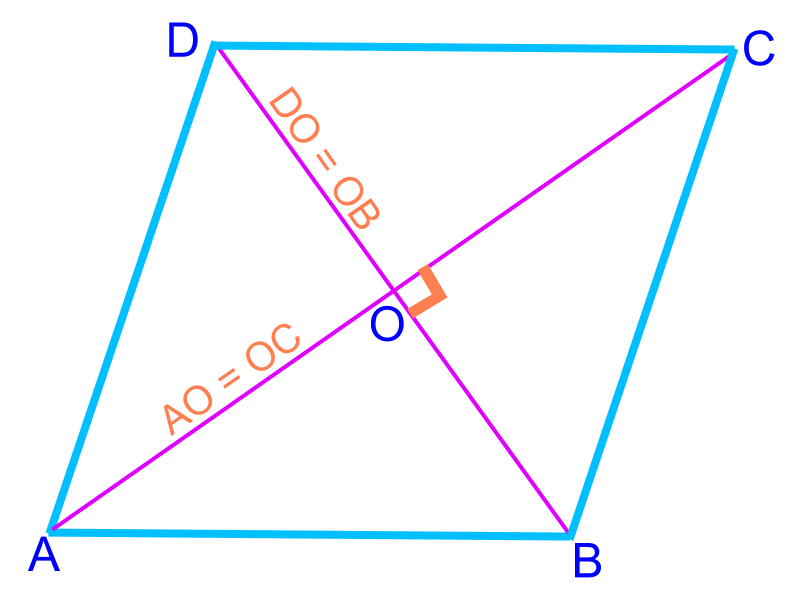A rhombus has the following properties.

•  All sides are equal (and parallel)

•  the diagonals perpendicularly bisect

•  opposite angles are equal

"Diagonals of rhombus perpendicularly bisect" This geometrical properties help to construct a perpendicular bisector to a line.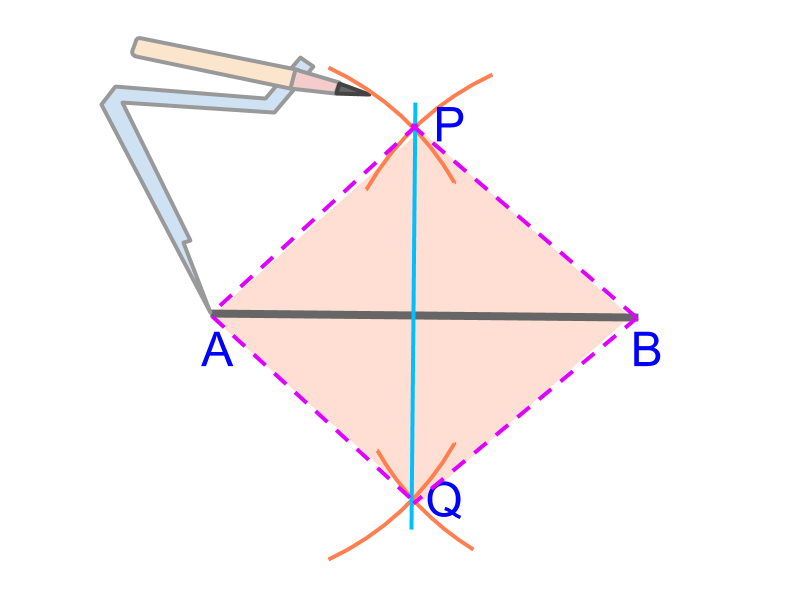Given line segment ¯¯¯¯¯¯AB$\overline{A B}$. The perpendicular bisector ¯¯¯¯pq$\overline{p q}$ is to be constructed.

•  Take a compass

•  fix a random distance between tips

•  construct arcs from position A$A$ above and below ¯¯¯¯¯¯AB$\overline{A B}$

•  construct arcs from position B$B$ above and below ¯¯¯¯¯¯AB$\overline{A B}$

•  the intersecting points are P$P$ and $Q$.

Note that the distance between tips of the compass is not modified and so, the sides $\overline{A P}$, $\overline{P B}$, $\overline{B Q}$, and $\overline{Q A}$ form a rhombus. From the property of a rhombus, the diagonals $\overline{A B}$ and $\overline{P Q}$ perpendicularly bisect each other.

Note for curious students: It can be a kite, with $\overline{A B}$ as minor diagonal. Property of a kite, the major diagonal $\overline{P Q}$ bisects minor diagonal $\overline{A B}$.

Bisecting a Line Segment : Use a compass to mark a rhombus with the given line segment as one of the diagonals. The perpendicular bisector is the other diagonal.

Perpendicular through a point on a line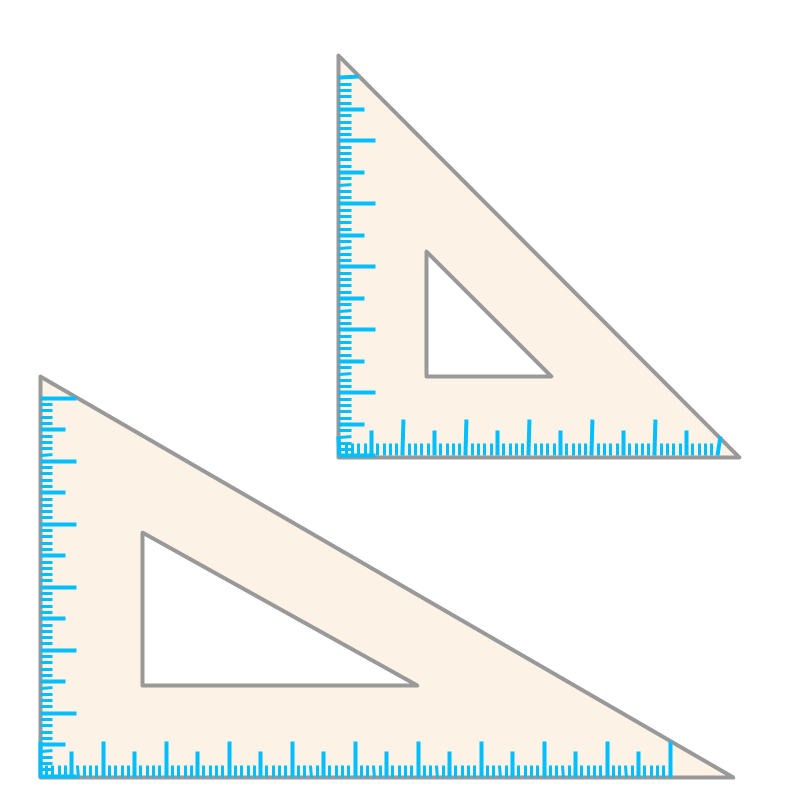Set squares or set triangles have sides or edges that are perpendicular.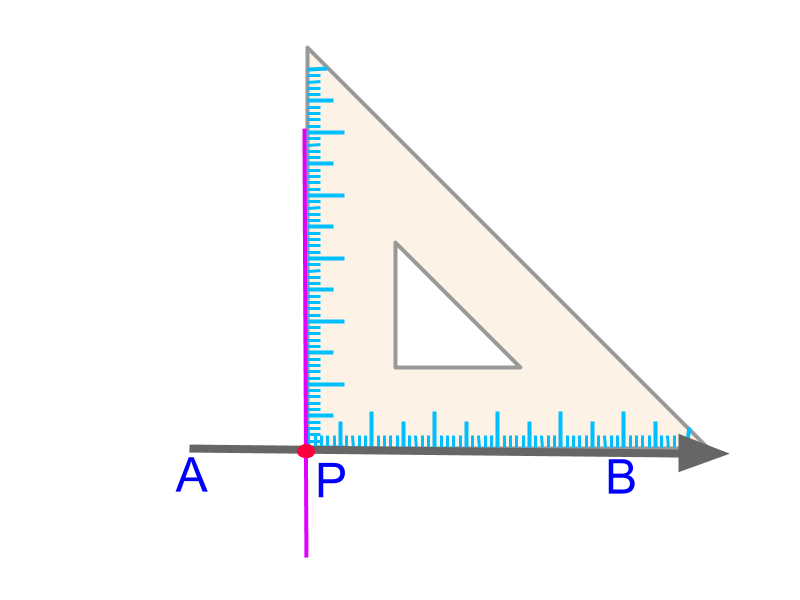Given line $\overline{A B}$ and a point $P$ on the line. Place the vertex of perpendicular edges of a set-square with one edge on the given line $\overline{A B}$. Construct the line along the other perpendicular edge of the set-square.

Perpendicular through a point on a line : Use the perpendicular edges of a set square, one edge on the given line and the perpendicular-vertex on the given point. Construct a line along the other perpendicular edge.

Some alternate methods can be used to construct a perpendicular through a point on a line.

•   method to construct perpendicular bisector using a compass

•   method to measure ${90}^{\circ}$ angle using a protractor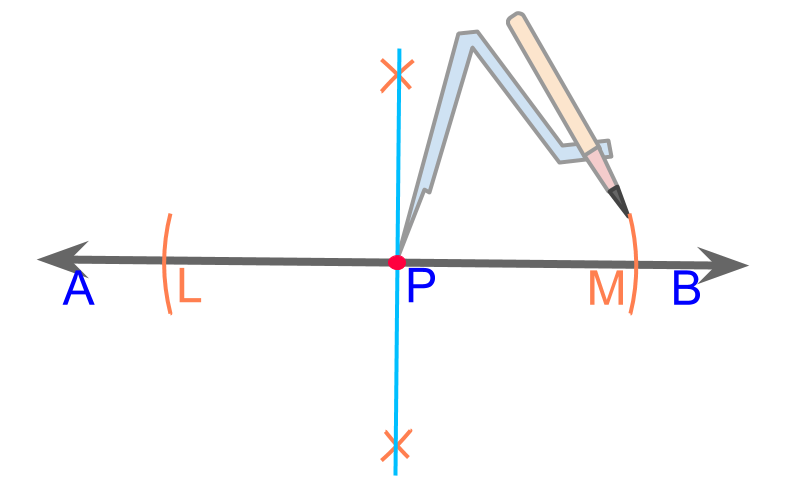Perpendicular through a point on a line : Use a compass to mark points $L$ and $M$ on the line that are in equal distance from the given point $P$. Construct the perpendicular bisector of $\overline{L M}$.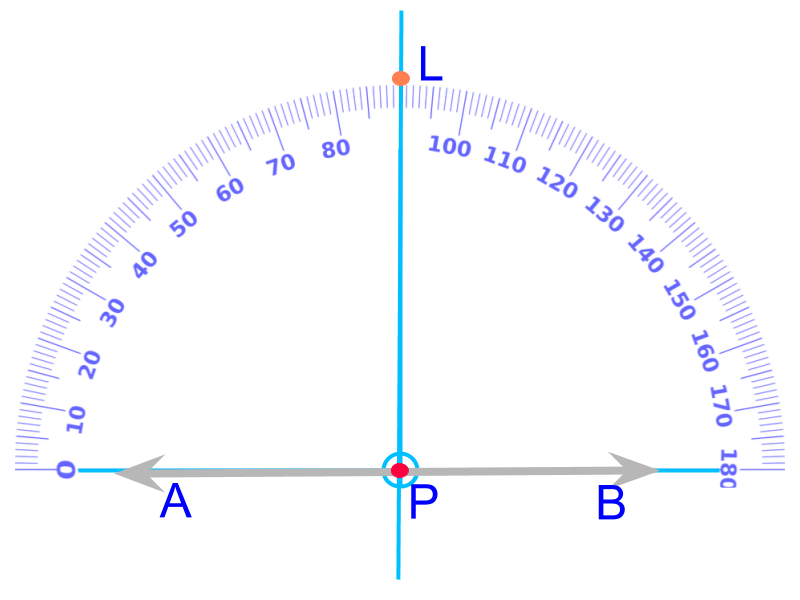Perpendicular through a point on a line : Place the protractor with origin on the given point and the base-line on the given line. Mark a point on ${90}^{\circ}$ angle and draw a line through the given point and the marked point.

Perpendicular to a point not on a line

Set squares can help to construct a perpendicular to a line through a point outside the line.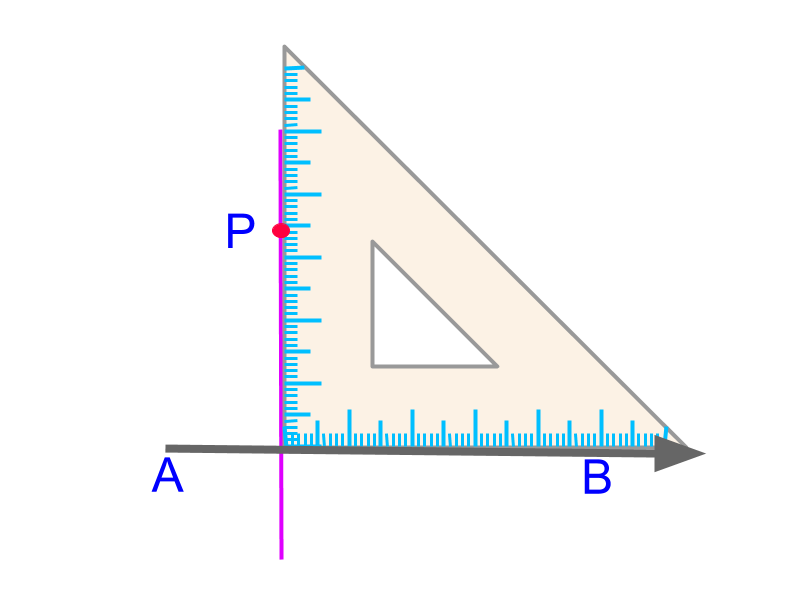Perpendicular to a Point : Use the perpendicular edges of a set square, one edge on the given line and the other edge on the given point. Construct a line along the other perpendicular edge.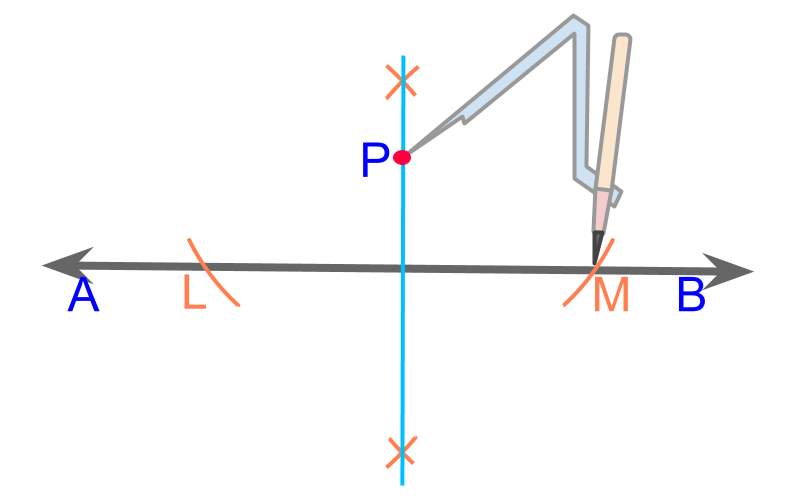Perpendicular to a Point : Use a compass to mark points $L$ and $M$ on the line that are in equal distance from the given point $P$. Construct the perpendicular bisector of $\overline{L M}$.

Bisecting an angle

A kite is a quadrilateral with two pair of equal and adjacent sides.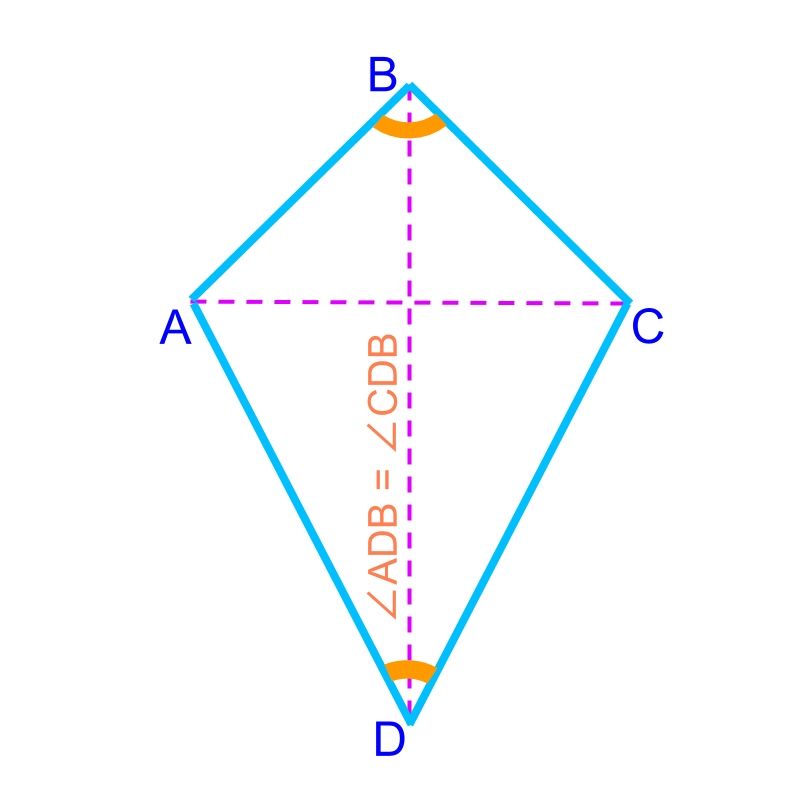A kite has the following properties

•  two pair of equal sides

•  major diagonal perpendicularly bisects the minor diagonal. the diagonal that divides the kite into two congruent triangles is called major. The other diagonal is the minor diagonal.

•  major diagonal bisects the angles at the vertices

•  two equal opposite angles and two unequal opposite angles -- all sum up to ${360}^{\circ}$

The geometrical property "major diagonal of a kite bisect the angles at both vertices" can be used to bisect a given angle.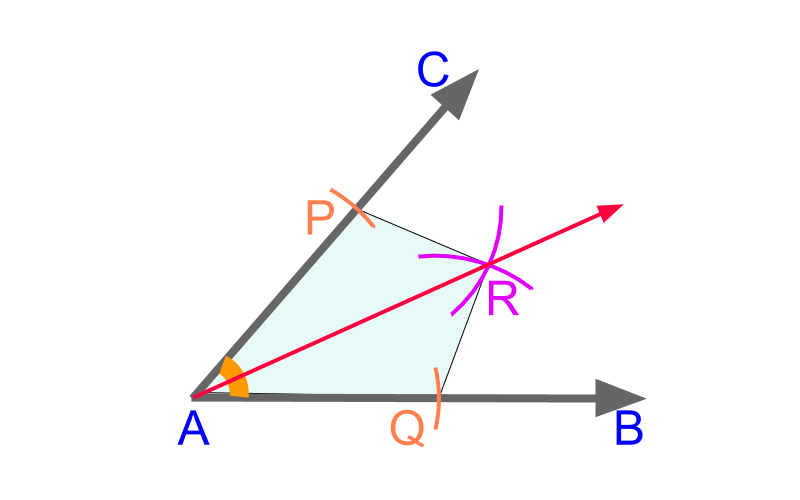Given angle $\angle B A C$, a kite $A Q R P$ is formed.

•  With an arbitrary measure on compass, mark points $P$ and $Q$ from the point $A$.

•  With another arbitrary measure on compass, construct arcs from points $P$ and $Q$. These two arcs cut at point $R$.

The quadrilateral $A Q R P$ is a kite and the line $\overline{A R}$ is the major bisector of angle $\angle B A C$.

Bisecting an Angle : Using the two rays of the angle, create a kite such that the major diagonal bisects the angle.

summary

Bisecting a Line Segment : Use a compass to mark a rhombus with the given line segment as one of the diagonals. The perpendicular bisector is the other diagonal.

Perpendicular through a point on a line : Use the perpendicular edges of a set square, one edge on the given line and the perpendicular-vertex on the given point. Construct a line along the other perpendicular edge.

Perpendicular through a point on a line : Use a compass to mark two points on the line that are in equal distance from the given point. Construct the perpendicular bisector between the two marked points.

Perpendicular through a point on a line : Place the protractor with origin on the given point and the base-line on the given line. Mark a point on ${90}^{\circ}$ angle and draw a line through the given point and the marked point.

Perpendicular to a Point : Use the perpendicular edges of a set square, one edge on the given line and the other edge on the given point. Construct a line along the other perpendicular edge.

Perpendicular to a Point : Use a compass to mark points two points on the line that are in equal distance from the given point. Construct the perpendicular bisector between the two marked points.

Bisecting an Angle : Using the two rays of the angle, create a kite such that the major diagonal bisects the angle.

Outline